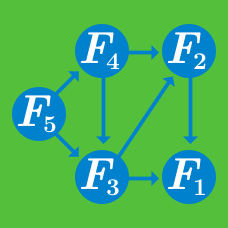Computer Science

# Egg Dropping

Consider the standard 2-egg problem. We wish to determine the critical height at which an egg breaks. That is we assume that there is some critical height $C$, such that if we drop an egg from height $h \leq C$ the egg does not break where as it will always break if dropped from height $h > C$. We want to minimize the number of trials and we are only given two eggs.

Let us consider the worst case scenario. Let $W(k)$ be the minimum number of trials required to identify $C$ under the worst case scenario given that we have two eggs and a building a height $k$.

How many values of $k > 0$ satisfy $W(k) = 18518505$?

Consider the classic egg drop problem. Let $S(n,k)$ be the minimum number of egg droppings that will suffice to find the critical floor in an $n$-story building given $k$ eggs.

$S(k,n)$ can be described as follows for an elementary function of $n$, $f(n)$.

$S(k,n) = \Theta(f(n,k))$

The function $f(n,k)$ is given by which of the following?

Consider the standard egg dropping problem, and design an algorithm that computes the maximum number of trials required to find the critical floor in the worst case. Find the maximum number of trials required in the worst case when you have three eggs and $105$ floors to check.

×